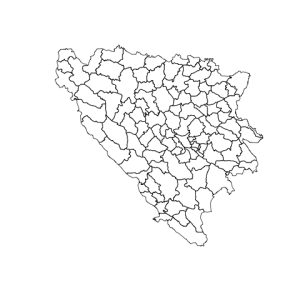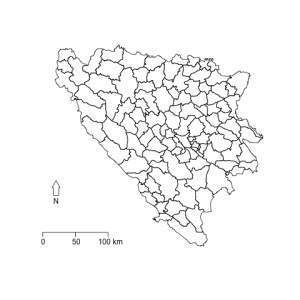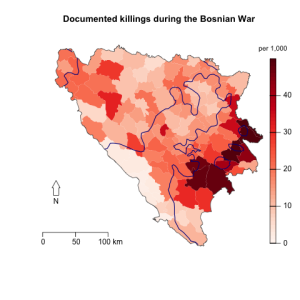# Andreas Beger

Data/political scientist

# Scale and north arrow for maps in R

Tags: #Bosnia #map scale #north arrow #R

A few months ago I produced some thematic maps of Bosnia (paper) using `maptools` and other packages in R, but I didn’t include scales or a north arrow. It sounds simple and `sp` has functions for doing those things, but I couldn’t get it to work well with my maps. Here is a basic map of Bosnia’s pre-war municipalities:

``````library(maptools)

plot(bosnia)
``````The function `map.scale()` from the `maps` package adds a scale. The position is in map units, latitude/longitude in this case:

``````library(maps)
map.scale(x=15.5, y=42.75, ratio=FALSE, relwidth=0.2)
``````

And `GISTools` `north.arrow` for the north arrow. Units are also in map units. This package has a map scale function as well, which looks nicer but is a little bit more complicated to set up.

``````library(GISTools)
north.arrow(xb=15.75, yb=43.25, len=0.05, lab="N")
``````

This will produce the following map:Adding these to a function I wrote for producing thematic maps of Bosnia produces this pretty nice map (with scale and north arrow!) of document per capita civil war deaths:Here is the function (which is pretty specific to the data I use):

``````ThematicMap <- function(vector, breaks, title, legend) {
require(maptools)
require(shape)
require(RColorBrewer)
require(GISTools)
require(maps)

plotvar <- unlist(vector)
nclr <- 9
plotclr <- brewer.pal(nclr, "Reds")
fillRed <- colorRampPalette(plotclr)
plotvar[plotvar >= maxy] <- maxy -1
colcode <- fillRed(maxy)[round(plotvar) + 1]
plot(bosnia, col = colcode, lty = 0, border = "gray")
plot(bosnia.st, add=TRUE, lwd=1, border = "gray30")
plot(bosnia.front93, add = TRUE, lty="solid", lwd=1.5, col="darkblue")
map.scale(x=15.5, y=42.75, relwidth=0.2, ratio=FALSE)
north.arrow(xb=15.75, yb=43.25, len=0.05, lab="N")
title(main = title)
colorlegend(posy = c(0.05,0.9), posx = c(0.9,0.92),
col = fillRed(maxy),
zlim=c(0, maxy), zval = breaks,
main = legend,
main.cex = 0.9)
par(bg='white')
}

killed.pk <- (killed/pop91*1000)
maxy <- 50
breaks <- c(0, 10, 20, 30, 40, Inf)